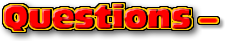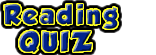ChaptersThis text is meant to accompany class discussions. It is not everything there is to know about uniform circular motion. It is meant as a  prep for class. More detailed notes and examples are given in the class notes, presentations, and demonstrations (click here.)Click for the questions that go with this reading

 Kinematics and Free Fall

This video can be found on YouTube at, http://www.youtube.com/watch?v=763d41g626k

 One more implied given for free fall. Look at the animation to the left. Notice how the "v," velocity, disappears. That is because it is zero at the highest point. The point in motion that is the farthest from the center of the planet is called "apogee." In the projectile motion the highest point of the motion when the vertical velocity is zero is called, "apogee." A word problem might say something like: "A ball is tossed up at 5 m/s. How much time will it take to reach the highest point?" Or is might say, "A ball is tossed up at 5 m/s. How high is the highest point of the motion?" Or even, ""A ball is tossed up at 5 m/s. How much time will it take to reach the apogee?" Then end of the ball's motion is at apogee. At apogee the vertical velocity is zero.
 Here's one more given that you may not have considered. Whan an object lands at the same height it is thrown from, the xo and x are both ZERO. The final velocity when it hits the ground is the negative of the initial velocity.Example w Free Fall
• Question
• Numerical Answer
• Video Solution
• Your Paper
A ball is tossed up at 5 m/s. How much time will it take to reach the highest point?
 0.51 seconds
 This video can be found on YouTube at http://youtu.be/vbTAAgrorfI
 This is what your paper should look like. Notice it is organized in column that can be read like reading a magazine article. The "givens" are listed with variable, number and units. What you are trying to do with the equation is shown and the equation appears with only variables, then numbers and units followed by a little math and the answer with units.by Tony Wayne ...(If you are a teacher, please feel free to use these resources in your teaching.)